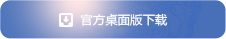立即扫码下载游戏

# 爆升100万战力，《天龙3D》美人阵法实用攻略

### 【美人阵法基础知识】

90级以上就能开放所有美人阵法，共计9个，各个阵法的属性加成效果如下：### 钟灵，木婉清，阿朱，阿紫，阿碧，阮星竹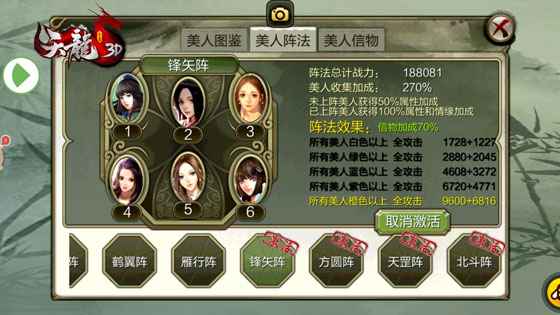### 王语嫣，西夏公主，李秋水，天山童姥，神仙姐姐，大理淑妃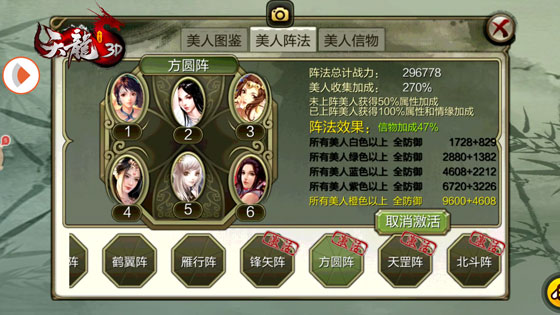### 梅剑，兰剑，竹剑，菊剑，辽国萧后，慕容夫人，萧夫人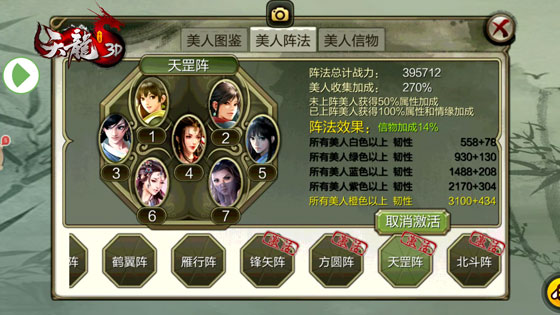### 娇俏钟灵，痴情婉清，绝色语嫣，温婉阿朱，刁蛮阿紫，倾城梦姑，妩媚青萝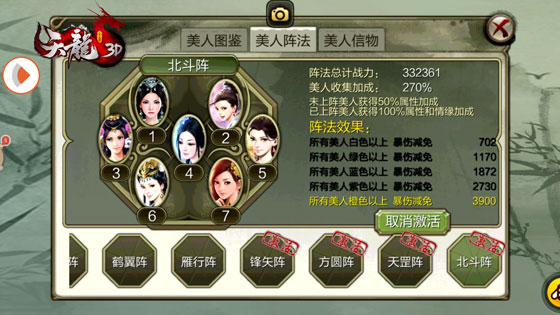### 【属性加成总计】

954+954+1192+954+596+1192+1192+1192+1192+2865+2865+2579+2579+2579+2579+2865+2865+2865+1433+2579+2292+2865+1433=44661

605+756+605+378+756+756+1820+1638+1638+1638+1638+1820+1820+1820+910+1456+1820+1274+910=24058

6.4%+8%+6.4%+8%+8%+8%+8%+8%+8%=68.8%

336+168=504

336

168

188081+296778+395712+332361=1212932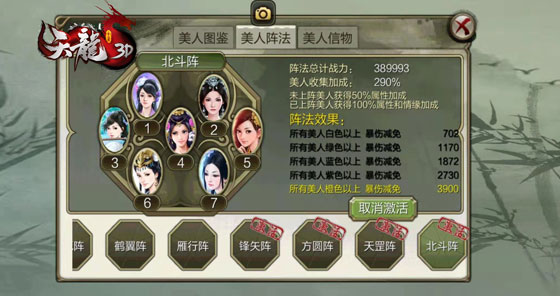### 【关于《天龙3D》】

ios下载地址>>>### TLBB3D